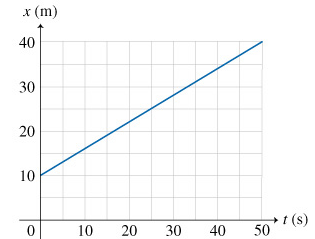# Problem: Part a. What is the overall displacement Δx of the particle?Part b. What is the average velocity vav of the particle over the time interval Δt = 50.0s?Part c. What is the instantaneous velocity v of the particle at t=10.0s?

###### FREE Expert Solution

Part a

Δx = xf - xi = 40 - 10 = 30 m

91% (456 ratings)###### Problem DetailsPart a. What is the overall displacement Δx of the particle?

Part b. What is the average velocity vav of the particle over the time interval Δt = 50.0s?

Part c. What is the instantaneous velocity v of the particle at t=10.0s?